# Tag: Brauer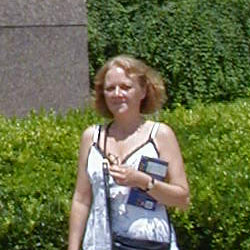This morning, Esther Beneish
arxived the paper The center of the generic algebra of degree p that may contain the most
significant advance in my favourite problem for over 15 years! In it she
claims to prove that the center of the generic division algebra of
degree p is stably rational for all prime values p. Let me begin by
briefly explaining what the problem is all about. Consider one n by n
matrix A which is sufficiently general, then it will have all its
eigenvalues distinct, but then it is via the Jordan normal form theorem uniquely
determined upto conjugation (that is, base change) by its
characteristic polynomial. In
other words, the conjugacy class of a sufficiently general n by n matrix
depends freely on the coefficients of the characteristic polynomial
(which are the n elementary symmetric functions in the eigenvalues of
the matrix). Now what about couples of n by n matrices (A,B) under
simultaneous conjugation (that is all couples of the form $~(g A g^{-1}, g B g^{-1})$ for some invertible n by n matrix g) ??? So,
does there exist a sort of Jordan normal form for couples of n by n
matrices which are sufficiently general? That is, are there a set of
invariants for such couples which determine it is freely upto
simultaneous conjugation?For couples of 2 by 2 matrices, Claudio Procesi rediscovered an old
result due to James Sylvester saying
that this is indeed the case and that the set of invariants consists of
the five invariants Tr(A),Tr(B),Det(A),Det(B) and Tr(AB). Now, Claudio
did a lot more in his paper. He showed that if you could prove this for
couples of matrices, you can also do it for triples, quadruples even any
k-tuples of n by n matrices under simultaneous conjugation. He also
related this problem to the center of the generic division algebra of
degree n (which was introduced earlier by Shimshon Amitsur in a rather
cryptic manner and for a while he simply refused to believe Claudio’s
description of this division algebra as the one generated by two
_generic_ n by n matrices, that is matrices filled with independent
variables). Claudio also gave the description of the center of this
algebra as a field of lattice-invariants (over the symmetric group S(n)
) which was crucial in subsequent investigations. If you are interested
in the history of this problem, its connections with Brauer group
problems and invariant theory and a short description of the tricks used
in proving the results I’ll mention below, you might have a look at the
talk Centers of Generic Division Algebras, the rationality problem 1965-1990
I gave in Chicago in 1990.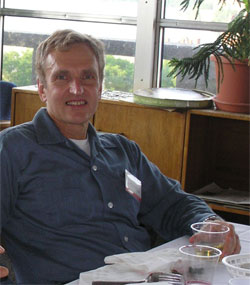The case of couples of 3 by 3 matrices was finally
settled in 1979 by Ed Formanek and a
year later he was able to solve also the case of couples of 4 by 4
matrices in a fabulous paper. In it, he used solvability of S(4) in an
essential way thereby hinting at the possibility that the problem might
no longer have an affirmative answer for larger values of n. When I read
his 4×4 paper I believed that someone able to prove such a result must
have an awesome insight in the inner workings of matrices and decided to
dedicate myself to this problem the moment I would get a permanent
job… . But even then it is a reckless thing to do. Spending all of
your time to such a difficult problem can be frustrating as there is no
guarantee you’ll ever write a paper. Sure, you can find translations of
the problem and as all good problems it will have connections with other
subjects such as moduli spaces of vectorbundles and of quiver
representations, but to do the ‘next number’ is another matter.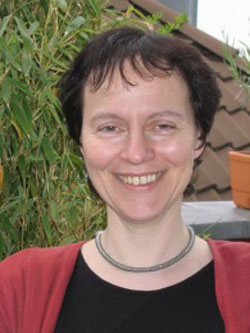Fortunately, early 1990, together with
Christine Bessenrodt we were
able to do the next two ‘prime cases’ : couples of 5 by 5 and couples of
7 by 7 matrices (Katsylo and Aidan Schofield had already proved that if
you could do it for couples of k by k and l by l matrices and if k and l
were coprime then you could also do it for couples of kl by kl matrices,
so the n=6 case was already done). Or did we? Well not quite, our
methods only allowed us to prove that the center is stably rational
that is, it becomes rational by freely adjoining extra variables. There
are examples known of stably rational fields which are NOT rational, but
I guess most experts believe that in the case of matrix-invariants
stable rationality will imply rationality. After this paper both
Christine and myself decided to do other things as we believed we had
reached the limits of what the lattice-method could do and we thought a
new idea was required to go further. If today’s paper by Esther turns
out to be correct, we were wrong. The next couple of days/weeks I’ll
have a go at her paper but as my lattice-tricks are pretty rusty this
may take longer than expected. Still, I see that in a couple of weeks
there will be a meeting in
Atlanta were Esther
and all experts in the field will be present (among them David Saltman
and Jean-Louis Colliot-Thelene) so we will know one way or the other
pretty soon. I sincerely hope Esther’s proof will stand the test as she
was the only one courageous enough to devote herself entirely to the
problem, regardless of slow progress.

Last time we saw
that a curve defined over $\overline{\mathbb{Q}}$ gives rise
to a permutation representation of $PSL_2(\mathbb{Z})$ or one
of its subgroups $\Gamma_0(2)$ (of index 2) or
$\Gamma(2)$ (of index 6). As the corresponding
monodromy group is finite, this representation factors through a normal
subgroup of finite index, so it makes sense to look at the profinite
completion
of $SL_2(\mathbb{Z})$, which is the inverse limit
of finite
groups $\underset{\leftarrow}{lim}~SL_2(\mathbb{Z})/N$
where N ranges over all normalsubgroups of finite index. These
profinte completions are horrible beasts even for easy groups such as
$\mathbb{Z}$. Its profinite completion
is

$\underset{\leftarrow}{lim}~\mathbb{Z}/n\mathbb{Z} = \prod_p \hat{\mathbb{Z}}_p$

where the right hand side
product of p-adic integers ranges over all prime numbers! The
_absolute Galois group_
$G=Gal(\overline{\mathbb{Q}}/\mathbb{Q})$ acts on all curves
defined over $\overline{\mathbb{Q}}$ and hence (via the Belyi
maps ans the corresponding monodromy permutation representation) there
is an action of $G$ on the profinite completions of the
carthographic groups.

This is what Grothendieck calls anabelian
algebraic geometry

Returning to the general
case, since finite maps can be interpreted as coverings over
$\overline{\mathbb{Q}}$ of an algebraic curve defined over
the prime field $~\mathbb{Q}$ itself, it follows that the
Galois group $G$ of $\overline{\mathbb{Q}}$ over
$~\mathbb{Q}$ acts on the category of these maps in a
natural way.
For instance, the operation of an automorphism
$~\gamma \in G$ on a spherical map given by the rational
function above is obtained by applying $~\gamma$ to the
coefficients of the polynomials P , Q. Here, then, is that
mysterious group $G$ intervening as a transforming agent on
topologico- combinatorial forms of the most elementary possible
nature, leading us to ask questions like: are such and such oriented
maps ‚conjugate or: exactly which are the conjugates of a given
oriented map? (Visibly, there is only a finite number of these).
I considered some concrete cases (for coverings of low degree) by
various methods, J. Malgoire considered some others ‚ I doubt that
there is a uniform method for solving the problem by computer. My
reflection quickly took a more conceptual path, attempting to
apprehend the nature of this action of G.
One sees immediately
that roughly speaking, this action is expressed by a certain
outer action of G on the profinite com- pactification of the
oriented cartographic group $C_+^2 = \Gamma_0(2)$ , and this
action in its turn is deduced by passage to the quotient of the
canonical outer action of G on the profinite fundamental group
$\hat{\pi}_{0,3}$ of
$(U_{0,3})_{\overline{\mathbb{Q}}}$ where
$U_{0,3}$ denotes the typical curve of genus 0 over the
prime field Q, with three points re- moved.
This is how my
attention was drawn to what I have since termed anabelian
algebraic geometry
, whose starting point was exactly a study
(limited for the moment to characteristic zero) of the action of
absolute Galois groups (particularly the groups Gal(K/K),
where K is an extension of finite type of the prime field) on
(profinite) geometric fundamental groups of algebraic varieties
(defined over K), and more particularly (break- ing with a
well-established tradition) fundamental groups which are very far
from abelian groups (and which for this reason I call
anabelian).
Among these groups, and very close to
the group $\hat{\pi}_{0,3}$ , there is the profinite
compactification of the modular group $Sl_2(\mathbb{Z})$,
whose quotient by its centre ±1 contains the former as congruence
subgroup mod 2, and can also be interpreted as an oriented
cartographic group, namely the one classifying triangulated
oriented maps (i.e. those whose faces are all triangles or
monogons).

and a bit further, on page
250

I would like to conclude this rapid outline
with a few words of commentary on the truly unimaginable richness
of a typical anabelian group such as $SL_2(\mathbb{Z})$
doubtless the most remarkable discrete infinite group ever
encountered, which appears in a multiplicity of avatars (of which
certain have been briefly touched on in the present report), and which
from the point of view of Galois-Teichmuller theory can be
considered as the fundamental ‚building block‚ of the
Teichmuller tower
The element of the structure of
$Sl_2(\mathbb{Z})$ which fascinates me above all is of course
the outer action of G on its profinite compactification. By
Bielyi’s theorem, taking the profinite compactifications of subgroups
of finite index of $Sl_2(\mathbb{Z})$, and the induced
outer action (up to also passing to an open subgroup of G), we
essentially find the fundamental groups of all algebraic curves (not
necessarily compact) defined over number fields K, and the outer
action of $Gal(\overline{K}/K)$ on them at least it is
true that every such fundamental group appears as a quotient of one
of the first groups.
Taking the anabelian yoga
(which remains conjectural) into account, which says that an anabelian
algebraic curve over a number field K (finite extension of Q) is
known up to isomorphism when we know its mixed fundamental group (or
what comes to the same thing, the outer action of
$Gal(\overline{K}/K)$ on its profinite geometric
fundamental group), we can thus say that
all algebraic
curves defined over number fields are contained in the profinite
compactification $\widehat{SL_2(\mathbb{Z})}$ and in the
knowledge of a certain subgroup G of its group of outer
automorphisms!

To study the absolute
Galois group $Gal(\overline{\mathbb{\mathbb{Q}}}/\mathbb{Q})$ one
investigates its action on dessins denfants. Each dessin will be part of
a finite family of dessins which form one orbit under the Galois action
and one needs to find invarians to see whether two dessins might belong
to the same orbit. Such invariants are called _Galois invariants_ and
quite a few of them are known.

Among these the easiest to compute
are

• the valency list of a dessin : that is the valencies of all
vertices of the same type in a dessin
• the monodromy group of a dessin : the subgroup of the symmetric group $S_d$ where d is
the number of edges in the dessin generated by the partitions $\tau_0$
and $\tau_1$ For example, we have seen
before
that the two
Mathieu-dessins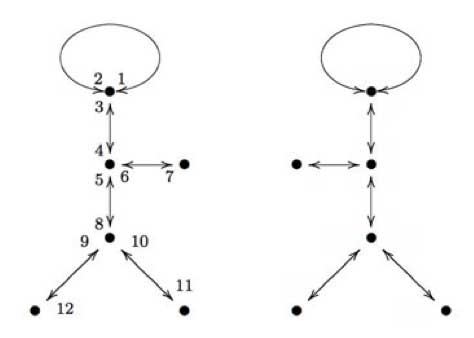form a Galois orbit. As graphs (remeber we have to devide each
of the edges into two and the midpoints of these halfedges form one type
of vertex, the other type are the black vertices in the graphs) these
are isomorphic, but NOT as dessins as we have to take the embedding of
them on the curve into account. However, for both dessins the valency
lists are (white) : (2,2,2,2,2,2) and (black) :
(3,3,3,1,1,1) and one verifies that both monodromy groups are
isomorphic to the Mathieu simple group $M_{12}$ though they are
not conjugated as subgroups of $S_{12}$.

Recently, new
Galois invariants were obtained from physics. In Children’s drawings
from Seiberg-Witten curves

the authors argue that there is a close connection between Grothendiecks
programme of classifying dessins into Galois orbits and the physics
problem of classifying phases of N=1 gauge theories…

Apart
from curves defined over $\overline{\mathbb{Q}}$ there are
other sources of semi-simple $SL_2(\mathbb{Z})$
representations. We will just mention two of them and may return to them
in more detail later in the course.

their representations
There are 26 exceptional finite simple groups
and as all of them are generated by two elements, there are epimorphisms
$\Gamma(2) \rightarrow S$ and hence all their representations
are also semi-simple $\Gamma(2)$-representations. In fact,
looking at the list of ‘standard generators’ of the sporadic
simples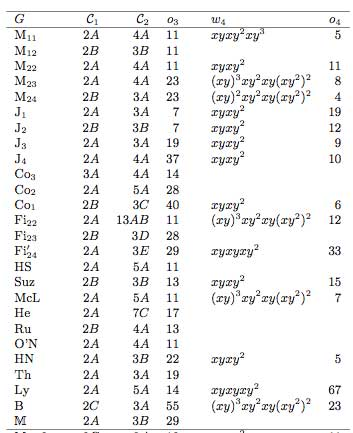(here the conjugacy classes of the generators follow the
notation of the Atlas project) we see that all but
possibly one are epimorphic images of $\Gamma_0(2) = C_2 \ast C_{\infty}$ and that at least 12 of then are epimorphic images
of $PSL_2(\mathbb{Z}) = C_2 \ast C_3$.

Rational conformal field theories Another
source of $SL_2(\mathbb{Z})$ representations is given by the
modular data associated to rational conformal field theories.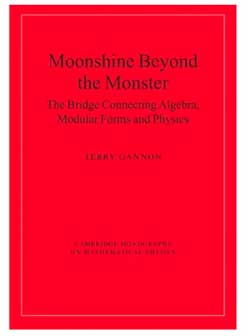These
representations also factor through a quotient by a finite index normal
subgroup and are therefore again semi-simple
$SL_2(\mathbb{Z})$-representations. For a readable
introduction to all of this see chapter 6 \”Modular group
representations throughout the realm\” of the
book Moonshine beyond the monster the bridge connecting algebra, modular forms and physics by Terry
Gannon
. In fact, the whole book
is a good read. It introduces a completely new type of scientific text,
that of a neverending survey paper…

Today we will explain how curves defined over
$\overline{\mathbb{Q}}$ determine permutation representations
of the carthographic groups. We have seen that any smooth projective
curve $C$ (a Riemann surface) defined over the algebraic
closure $\overline{\mathbb{Q}}$ of the rationals, defines a
_Belyi map_ $\xymatrix{C \ar[rr]^{\pi} & & \mathbb{P}^1}$ which is only ramified over the three points
$\\{ 0,1,\infty \\}$. By this we mean that there are
exactly $d$ points of $C$ lying over any other point
of $\mathbb{P}^1$ (we call $d$ the degree of
$\pi$) and that the number of points over $~0,1~$ and
$~\infty$ is smaller than $~d$. To such a map we
associate a _dessin d\’enfant_, a drawing on $C$ linking the
pre-images of $~0$ and $~1$ with exactly $d$
edges (the preimages of the open unit-interval). Next, we look at
the preimages of $~0$ and associate a permutation
$\tau_0$ of $~d$ letters to it by cycling
counter-clockwise around these preimages and recording the edges we
meet. We repeat this procedure for the preimages of $~1$ and
get another permutation $~\tau_1$. That is, we obtain a
subgroup of the symmetric group $\langle \tau_0,\tau_1 \rangle \subset S_d$ which is called the monodromy
group
of the covering $\pi$.For example, the
dessin on the right is
associated to a degree $8$ map $\mathbb{P}^1 \rightarrow \mathbb{P}^1$ and if we let the black (resp. starred) vertices be
the preimages of $~0$ (respectively of $~1$), then the
corresponding partitions are $\tau_0 = (2,3)(1,4,5,6)$
and $\tau_1 = (1,2,3)(5,7,8)$ and the monodromy group
is the alternating group $A_8$ (use
GAP ).

But wait! The map is also
ramified in $\infty$ so why don\’t we record also a
permutation $\tau_{\infty}$ and are able to compute it from
the dessin? (Note that all three partitions are needed if we want to
reconstruct $C$ from the $~d$ sheets as they encode in
which order the sheets fit together around the preimages). Well,
the monodromy group of a $\mathbb{P}^1$ covering ramified only
in three points is an epimorphic image of the fundamental
group
of the sphere
minus three points $\pi_1(\mathbb{P}^1 – { 0,1,\infty })$ That is, the group of all loops beginning and
ending in a basepoint upto homotopy (that is, two such loops are the
same if they can be transformed into each other in a continuous way
while avoiding the three points).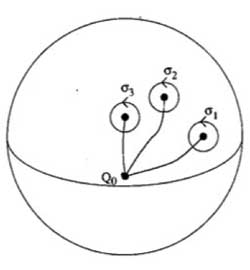This group is generated by loops
$\sigma_i$ running from the basepoint to nearby the i-th
point, doing a counter-clockwise walk around it and going back to be
basepoint $Q_0$ and the epimorphism to the monodromy group is given by sending

$\sigma_1 \mapsto \tau_0~\quad~\sigma_2 \mapsto \tau_1~\quad~\sigma_3 \mapsto \tau_{\infty}$

Now,
these three generators are not independent. In fact, this fundamental
group is

$\pi_1(\mathbb{P}^1 – \\{ 0,1,\infty \\}) = \langle \sigma_1,\sigma_2,\sigma_3~\mid~\sigma_1 \sigma_2 \sigma_3 = 1 \rangle$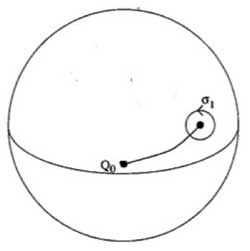To understand this, let us begin
with an easier case, that of the sphere minus one point. The fundamental group of the plane minus one point is
$~\mathbb{Z}$ as it encodes how many times we walk around the
point. However, on the sphere the situation is different as we can make
our walk around the point longer and longer until the whole walk is done
at the backside of the sphere and then we can just contract our walk to
the basepoint. So, there is just one type of walk on a sphere minus one
point (upto homotopy) whence this fundamental group is trivial. Next,
let us consider the sphere minus two points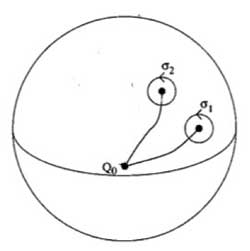Repeat the foregoing to the walk $\sigma_2$, that
is, strech the upper part of the circular tour all over the backside of
the sphere and then we see that we can move it to fit with the walk
$\sigma_1$ BUT for the orientation of the walk! That is, if we do this
modified walk $\sigma_1 \sigma_2^{\’}$ we just made the
trivial walk. So, this fundamental group is $\langle \sigma_1,\sigma_2~\mid~\sigma_1 \sigma_2 = 1 \rangle = \mathbb{Z}$ This is also the proof of the above claim. For,
we can modify the third walk $\sigma_3$ continuously so that
it becomes the walk $\sigma_1 \sigma_2$ but
with the reversed orientation ! As $\sigma_3 = (\sigma_1 \sigma_2)^{-1}$ this allows us to compute the
\’missing\’ permutation $\tau_{\infty} = (\tau_0 \tau_1)^{-1}$ In the example above, we obtain
$\tau_{\infty}= (1,2,6,5,8,7,4)(3)$ so it has two cycles
corresponding to the fact that the dessin has two regions (remember we
should draw ths on the sphere) : the head and the outer-region. Hence,
the pre-images of $\infty$ correspond to the different regions of the
dessin on the curve $C$. For another example,
consider the degree 168 map

$K \rightarrow \mathbb{P}^1$

which is the modified orbit map for the action of
$PSL_2(\mathbb{F}_7)$ on the Klein quartic.
The corresponding dessin is the heptagonal construction of the Klein
quartic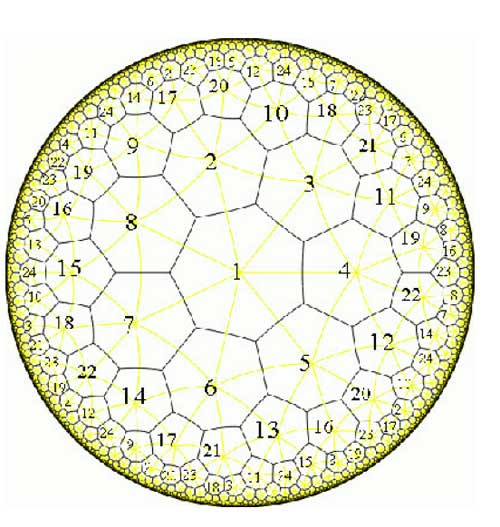Here, the pre-images of 1 correspond to the midpoints of the
84 edges of the polytope whereas the pre-images of 0 correspond to the
56 vertices. We can label the 168 half-edges by numbers such that
$\tau_0$ and $\tau_1$ are the standard generators b
resp. a of the 168-dimensional regular representation (see the atlas
page
).
Calculating with GAP the element $\tau_{\infty} = (\tau_0 \tau_1)^{-1} = (ba)^{-1}$ one finds that this permutation
consists of 24 cycles of length 7, so again, the pre-images of
$\infty$ lie one in each of the 24 heptagonal regions of the
Klein quartic. Now, we are in a position to relate curves defined
over $\overline{Q}$ via their Belyi-maps and corresponding
dessins to Grothendiecks carthographic groups $\Gamma(2)$,
$\Gamma_0(2)$ and $SL_2(\mathbb{Z})$. The
dessin gives a permutation representation of the monodromy group and
because the fundamental group of the sphere minus three
points $\pi_1(\mathbb{P}^1 – \\{ 0,1,\infty \\}) = \langle \sigma_1,\sigma_2,\sigma_3~\mid~\sigma_1 \sigma_2 \sigma_3 = 1 \rangle = \langle \sigma_1,\sigma_2 \rangle$ is the free group op two generators, we see that
any dessin determines a permutation representation of the congruence
subgroup $\Gamma(2)$ (see this
post
where we proved that this
group is free). A clean dessin is one for which one type of
vertex has all its valancies (the number of edges in the dessin meeting
the vertex) equal to one or two. (for example, the pre-images of 1 in
the Klein quartic-dessin or the pre-images of 1 in the monsieur Mathieu
example
) The corresponding
permutation $\tau_1$ then consists of 2-cycles and hence the
monodromy group gives a permutation representation of the free
product $C_{\infty} \ast C_2 = \Gamma_0(2)$ Finally, a clean dessin is said to be a
quilt dessin if also the other type of vertex has all its valancies
equal to one or three (as in the Klein quartic or Mathieu examples).
Then, the corresponding permutation has order 3 and for these
quilt-dessins the monodromy group gives a permutation representation of
the free product $C_2 \ast C_3 = PSL_2(\mathbb{Z})$ Next time we will see how this lead
Grothendieck to his anabelian geometric approach to the absolute Galois
group.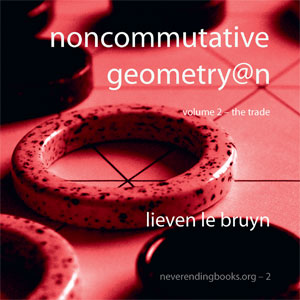Half a year
ago, it all started with NeverEndingBooks in
which I set out a rather modest goal :

Why NeverEndingBooks
? We all complain about exaggerated prices of mathematical books from
certain publishers, poor quality of editing and refereeing offered, as
well as far too stringent book-contracts. Rather than lamenting about
this, NeverEndingBooks gives itself one year to learn (and report) about
the many aspects of the book-production cycle and to explore whether an
alternative exists. If at the end of this year we will have produced at
least one book this experiment will be considered a success. If,
however, we find out that it is an impossible task, we will explain
where it all went wrong and why it is better to stick to an established
PublishingHouse and accept its terms.

I assume we did
manage to do it after all as you may check by visiting our storefront :
www.lulu.com/neverendingbooks
. However, it all turned out to be
quite different from what I had in mind half a year ago. So, perhaps
it’s time to recap.

Originally, I’d planned to partner-up
with the publisher-on-demand LightningSource but
in the process they did require a VAT-number. In Belgium, the safest
way to get one is to set up a non-profit organization (a VZW as we call
it). But then you have to write down your legal statutes, get them
published in the Moniteur
Belge
(at a hefty price) but what really put me off was that you
have to set up a “board of directors” consisting of at least three
people. I don‚Äôt mind following a folly but if I have to involve others
I usually pass, so I abandoned the whole idea. Still, I couldn‚Äôt help
talking about the VAT-problem and at a certain time there was an idea to
revive a sleeping VZW (=non-profit organization) of the Belgian Mathematical Society, the _MaRC_ (MAthematical Research Centre), the
statutes of which allowed to become a publishing house. But, this
wouldn‚Äôt involve just two other people but the whole BMS so I decided
to forget all about it and have a short vacation in France together with
a few (former)PhD-students.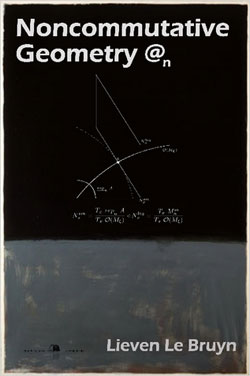Given
plenty of sun, cheese and whine (not necessarily in that order) sooner
or later we had to talk about _the_ problem. For Raf it was the
first time he heard about it but when we realized I thought one could
easily publish books well under 25 dollars he was immediately interested
and insisted we should set up a board of directors and continue with the
plan. The different roles to play in the board were more or less
self-evident : I had to be the trasurer (given the fact that I was the
only with a secure, though small, income), Geert had to become chairman
(being the only one possessing suits), Raf would be secretary (being the
only one who could write better Flemish than English) and Jan or Stijn would do PR (as they
are the only ones having enough social skills). So, we went back willing
to go through the whole process (at least 3 months) of obtaining a
VAT-number.

But then Raf got so interested in the whole idea
that he explored other possibilities (I think he was more motivated by
the fact that his sister wanted to publish her thesis rather than
anything else) and came up with lulu.com. No legal hassle, no
VAT-numbers, nothing required (or so it seemed). Still, before risking
his sister‚Äôs thesis he wanted to check the service out and as it is a
lot easier to take a book lying around rather than write one yourself he
took my version 2 and published it at
Lulu’s (since then this version is nicknamed Rothko@n).

Although I gave him the permission to do so, it didn‚Äôt feel right
that people should pay even a small amount for a nicely bound unedited
version 2. So, the last month and a half I‚Äôve been editing and
partially rewriting version 2 and the two volumes are now available! Major changes
are to the 4 middle chapters. There is now chapter 3 “Etale
Technology” which contains all of the etale tricks scattered earlier
in two chapters, chapter 4 ‚”Quiver Representations” collects all the
quiver material (again, scattered throughout the previous version).
Chapter 5 ‚”Semisimple Representations” now includes recent material
such as Raf’s characterization of the smooth locus of Cayley-smooth
orders and our (together with Geert) classification of the central
singularities, and chapter 6 ‚”Nilpotent Representations” now
includes the material on Brauer-Severi varities which was in version 1
but somehow didnt make it to version 2 before.One way to increase the blogshare-value of this site might be to
give readers more of what they want. In fact, there is an excellent
guide for those who really want to increase traffic on their site
called 26
Steps to 15k a Day
. A somewhat sobering suggestion is rule S :

“Think about what people want. They
they are coming to your site looking for “their
content”.”

But how do we know what
people want? Well, by paying attention to Google-referrals according
to rule U :

“The search engines will
tell you exactly what they want to be fed – listen closely, there is
gold in referral logs, it's just a matter of panning for
it.”

show over the last couple of days? Well, here are the top recent
key-words given to Google to get here :

13 :
carolyn dean jacobian conjecture
11 : carolyn dean jacobian

9 : brauer severi varieties
7 : latexrender

7 : brauer severi
7 : spinor bundles
7 : ingalls
azumaya
6 : [Unparseable or potentially dangerous latex
formula Error 6 ]
6 : jacobian conjecture carolyn dean

See a pattern? People love to hear right now about
the solution of the Jacobian conjecture in the plane by Carolyn Dean.
and it may take a while before you know why there is a photo of Tracy
Chapman next to this post…

First, it seems I only got
part of the Melvin Hochster
email
. Here is the final part I was unaware of (thanks to not even wrong)

Earlier papers established the following: if
there is
a counterexample, the leading forms of $f$ and $g$
may
be assumed to have the form $(x^a y^b)^J$ and $(x^a y^b)^K$,
where $a$ and $b$ are relatively prime and neither
$J$
nor $K$ divides the other (Abhyankar, 1977). It is known
that
$a$ and $b$ cannot both be $1$ (Lang, 1991) and that one
may
assume that $C[f,g]$ does not contain a degree one
polynomial
in $x, y$ (Formanek, 1994).

Let $D_x$ and $D_y$ indicate partial differentiation with respect

to $x$ and $y$, respectively. A difficult result of Bass (1989)

asserts that if $D$ is a non-zero operator that is a polynomial

over $C$ in $x D_x$ and $y D_y$, $G$ is in $C[x,y]$ and $D(G)$

is in $C[f,g]$, then $G$ is in $C[f,g]$.

The proof
proceeds by starting with $f$ and $g$ that give
a
counterexample, and recursively constructing sequences of
elements and derivations with remarkable, intricate and
surprising relationships. Ultimately, a contradiction is
obtained by studying a sequence of positive integers associated
with the degrees of the elements constructed. One delicate
argument shows that the sequence is bounded. Another delicate
argument shows that it is not. Assuming the results described
above, the proof, while complicated, is remarkably self-contained
and can be understood with minimal background in algebra.

• Mel Hochster

conjecture-post at not even wrong and
the discussion in the comments to it : there were a few instances I
really wanted to join in but I'll do it here. To begin, I was a
bit surprised of the implicit attack in the post

Dean hasn't published any papers in almost 15 years and is
nominally a lecturer in mathematics education at Michigan.

But this was immediately addressed and retracted in

Just curious. What exactly did
you mean by “nominally a lecturer”?
Posted by mm
at November 10, 2004 10:54 PM

I don't know
anything about Carolyn Dean personally, just that one place on the
Michigan web-site refers to her as a “lecturer”, another
as a “visiting lecturer”. As I'm quite well aware from
personal experience, these kinds of titles can refer to all sorts of
different kinds of actual positions. So the title doesn't tell you
much, which is what I was awkwardly expressing.
Posted by Peter
at November 10, 2004 11:05 PM

Well, I know a few things
about Carolyn Dean personally, the most relevant being that she is a
very careful mathematician. I met her a while back (fall of 1985) at
UCSD where she was finishing (or had finished) her Ph.D. If Lance
Small's description of me would have been more reassuring, we
might even have ended up sharing an apartment (quod non). Instead I
ended up with Claudio
Procesi
… Anyway, it was a very enjoyable month with a group
of young starting mathematicians and I fondly remember some
dinner-parties we organized. The last news I heard about Carolyn was
10 to 15 years ago in Oberwolfach when it was rumoured that she had
solved the Jacobian conjecture in the plane… As far as I recall,
the method sketched by Hochster in his email was also the one back
then. Unfortunately, at the time she still didn't have all pieces
in place and a gap was found (was it by Toby Stafford? or was it
Hochster?, I forgot). Anyway, she promptly acknowledged that there was
a gap.
At the time I was dubious about the approach (mostly
because I was secretly trying to solve it myself) but today my gut
feeling is that she really did solve it. In recent years there have
been significant advances in polynomial automorphisms (in particular
the tame-wild problem) and in the study of the Hilbert scheme of
points in the plane (which I always thought might lead to a proof) so
perhaps some of these recent results did give Carolyn clues to finish
off her old approach? I haven't seen one letter of the proof so
I'm merely speculating here. Anyway, Hochster's assurance that
the proof is correct is good enough for me right now.
Another
discussion in the NotEvenWrong-comments was on the issue that several
old problems were recently solved by people who devoted themselves for
several years solely to that problem and didn't join the parade of
dedicated follower of fashion-mathematicians.

It is remarkable that the last decade has seen great progress in
math (Wiles proving Fermat's Last Theorem, Perelman proving the
Poincare Conjecture, now Dean the Jacobian Conjecture), all achieved
by people willing to spend 7 years or more focusing on a single
problem. That's not the way academic research is generally
structured, if you want grants, etc. you should be working on much
shorter term projects. It's also remarkable that two out of three
of these people didn't have a regular tenured position.

I think particle theory should learn from this. If
some of the smarter people in the field would actually spend 7 years
concentrating on one problem, the field might actually go somewhere
Posted by Peter at November
13, 2004 08:56 AM

Here we come close to a major problem of
today's mathematics. I have the feeling that far too few
mathematicians dedicate themselves to problems in which they have a
personal interest, independent of what the rest of the world might
think about these problems. Far too many resort to doing trendy,
technical mathematics merely because it is approved by so called
'better' mathematicians. Mind you, I admit that I did fall in
that trap myself several times but lately I feel quite relieved to be
doing just the things I like to do no matter what the rest may think
about it. Here is a little bit of advice to some colleagues : get
yourself an iPod and take
some time to listen to songs like this one :

Don't be tempted by the shiny apple
Don't you eat
of a bitter fruit
Hunger only for a taste of justice

Hunger only for a world of truth
'Cause all that you have